# sbiofitmixed

Fit nonlinear mixed-effects model (requires Statistics and Machine Learning Toolbox software)

## Syntax

``fitResults = sbiofitmixed(sm,grpData,ResponseMap,covEstiminfo)``
``fitResults = sbiofitmixed(sm,grpData,ResponseMap,covEstiminfo,dosing)``
``fitResults = sbiofitmixed(sm,grpData,ResponseMap,covEstiminfo,dosing,functionName)``
``fitResults = sbiofitmixed(sm,grpData,ResponseMap,covEstiminfo,dosing,functionName,opt)``
``fitResults = sbiofitmixed(sm,grpData,ResponseMap,covEstiminfo,dosing,functionName,opt,variants)``
``fitResults = sbiofitmixed(___,Name,Value)``
``````[fitResults,simDataI,simDataP] = sbiofitmixed(_)``````

## Description

example

````fitResults = sbiofitmixed(sm,grpData,ResponseMap,covEstiminfo)` performs nonlinear mixed-effects estimation using the SimBiology® model `sm` and returns a `NLMEResults` object `fitResults`. `grpData` is a ```groupedData object``` specifying the data to fit. `ResponseMap` defines the mapping between the model components and response data in `grpData`. `covEstiminfo` is a `CovariateModel` object or an array of `estimatedInfo` objects that defines the parameters to be estimated.If the model contains active doses and variants, they are applied during the simulation.```

example

````fitResults = sbiofitmixed(sm,grpData,ResponseMap,covEstiminfo,dosing)` uses the dosing information specified by a matrix of SimBiology dose objects `dosing` instead of using the active doses of the model `sm` if there are any.```

example

````fitResults = sbiofitmixed(sm,grpData,ResponseMap,covEstiminfo,dosing,functionName)` uses the estimation function specified by `functionName` that must be either `'nlmefit'` or `'nlmefitsa'`.```

example

````fitResults = sbiofitmixed(sm,grpData,ResponseMap,covEstiminfo,dosing,functionName,opt)` uses the additional options specified by `opt` for the estimation function `functionName`.```

example

````fitResults = sbiofitmixed(sm,grpData,ResponseMap,covEstiminfo,dosing,functionName,opt,variants)` applies variant objects specified as `variants` instead of using any active variants of the model.```
````fitResults = sbiofitmixed(___,Name,Value)` uses additional options specified by one or more name-value arguments.```
``````[fitResults,simDataI,simDataP] = sbiofitmixed(_)``` returns a vector of results objects `fitResults`, vector of simulation results `simDataI` using individual-specific parameter estimates, and vector of simulation results `simDataP` using population parameter estimates. Note`sbiofitmixed` unifies `sbionlmefit` and `sbionlmefitsa` estimation functions. Use `sbiofitmixed` to perform nonlinear mixed-effects modeling and estimation.`sbiofitmixed` simulates the model using a `SimFunction object`, which automatically accelerates simulations by default. Hence it is not necessary to run `sbioaccelerate` before you call `sbiofitmixed`. ```

## Examples

collapse all

This example uses data collected on 59 preterm infants given phenobarbital during the first 16 days after birth . Each infant received an initial dose followed by one or more sustaining doses by intravenous bolus administration. A total of between 1 and 6 concentration measurements were obtained from each infant at times other than dose times, for a total of 155 measurements. Infant weights and APGAR scores (a measure of newborn health) were also recorded.

`load pheno.mat ds`

Convert the dataset to a `groupedData object`, a container for holding tabular data that is divided into groups. It can automatically identify commonly used variable names as the grouping variable or independent (time) variable. Display the properties of the data and confirm that `GroupVariableName` and `IndependentVariableName` are correctly identified as `'ID'` and `'TIME'`, respectively.

```data = groupedData(ds); data.Properties```
```ans = struct with fields: Description: '' UserData: [] DimensionNames: {'Observations' 'Variables'} VariableNames: {'ID' 'TIME' 'DOSE' 'WEIGHT' 'APGAR' 'CONC'} VariableDescriptions: {} VariableUnits: {} VariableContinuity: [] RowNames: {} CustomProperties: [1×1 matlab.tabular.CustomProperties] GroupVariableName: 'ID' IndependentVariableName: 'TIME' ```

Create a simple one-compartment PK model with bolus dosing and linear clearance to fit such data. Use the `PKModelDesign` object to construct the model. Each compartment is defined by a name, dosing type, a clearance type, and whether or not the dosing requires a lag parameter. After constructing the model, you can also get a `PKModelMap` object `map` that lists the names of species and parameters in the model that are most relevant for fitting.

```pkmd = PKModelDesign; addCompartment(pkmd,'Central','DosingType','Bolus',... 'EliminationType','linear-clearance',... 'HasResponseVariable',true,'HasLag',false); [onecomp, map] = pkmd.construct;```

Describe the experimentally measured response by mapping the appropriate model component to the response variable. In other words, indicate which species in the model corresponds to which response variable in the data. The `PKModelMap` property `Observed` indicates that the relevant species in the model is `Drug_Central`, which represents the drug concentration in the system. The relevant data variable is `CONC`, which you visualized previously.

`map.Observed`
```ans = 1×1 cell array {'Drug_Central'} ```

Map the `Drug_Central` species to the `CONC` variable.

`responseMap = 'Drug_Central = CONC';`

The parameters to estimate in this model are the volume of the central compartment `Central` and the clearance rate `Cl_Central`. The `PKModelMap` property `Estimated` lists these relevant parameters. The underlying algorithm of `sbiofit` assumes parameters are normally distributed, but this assumption may not be true for biological parameters that are constrained to be positive, such as volume and clearance. Specify a log transform for the estimated parameters so that the transformed parameters follow a normal distribution. Use an `estimatedInfo` object to define such transforms and initial values (optional).

`map.Estimated`
```ans = 2×1 cell {'Central' } {'Cl_Central'} ```

Define such estimated parameters, appropriate transformations, and initial values.

`estimatedParams = estimatedInfo({'log(Central)','log(Cl_Central)'},'InitialValue',[1 1]);`

Each infant received a different schedule of dosing. The amount of drug is listed in the data variable `DOSE`. To specify these dosing during fitting, create dose objects from the data. These objects use the property `TargetName` to specify which species in the model receives the dose. In this example, the target species is `Drug_Central`, as listed by the `PKModelMap` property `Dosed`.

`map.Dosed`
```ans = 1×1 cell array {'Drug_Central'} ```

Create a sample dose with this target name and then use the `createDoses` method of `groupedData` object `data` to generate doses for each infant based on the dosing data `DOSE`.

```sampleDose = sbiodose('sample','TargetName','Drug_Central'); doses = createDoses(data,'DOSE','',sampleDose);```

Fit the model.

`[nlmeResults,simI,simP] = sbiofitmixed(onecomp,data,responseMap,estimatedParams,doses,'nlmefit');`

Visualize the fitted results using individual-specific parameter estimates.

`plot(nlmeResults,'ParameterType','individual');`Visualize the fitted results using population parameter estimates.

`plot(nlmeResults,'ParameterType','population');`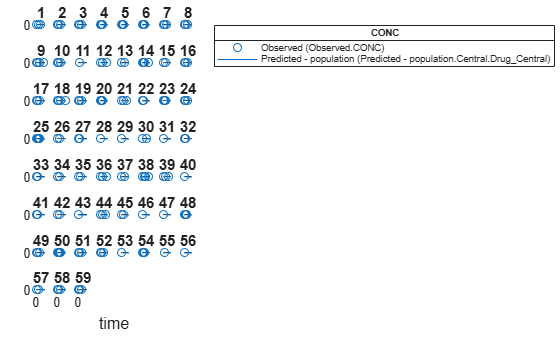Display the variation of estimated parameters using boxplot.

`boxplot(nlmeResults)`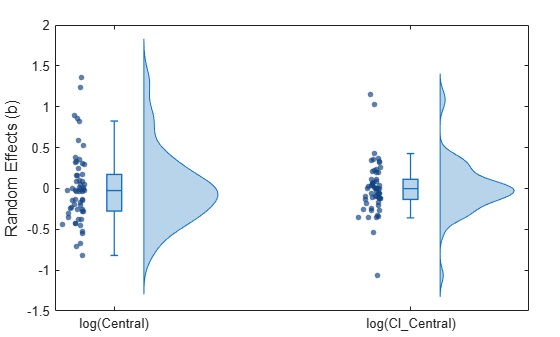Compare the model predictions to the actual data.

`plotActualVersusPredicted(nlmeResults)`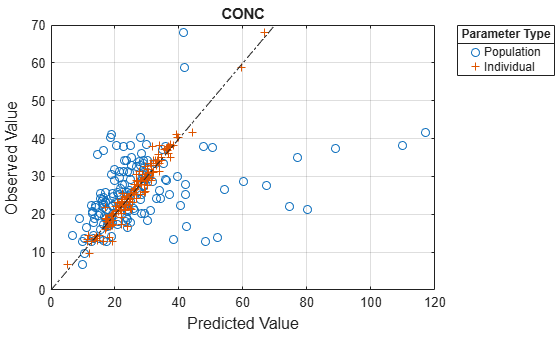Plot the distribution of residuals.

`plotResidualDistribution(nlmeResults)`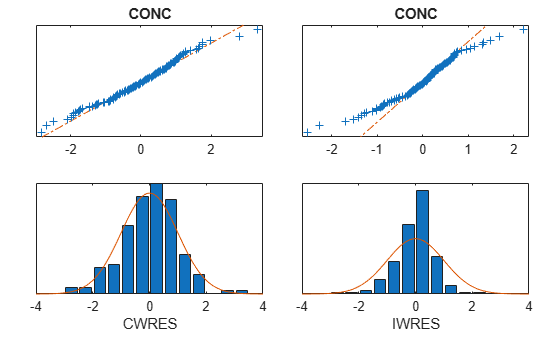Plot residuals for each response using the model predictions on x-axis.

`plotResiduals(nlmeResults,'Predictions')`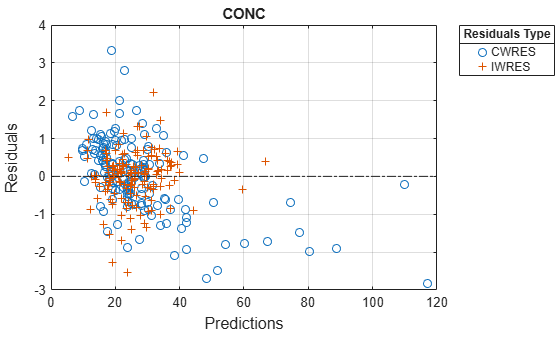## Input Arguments

collapse all

SimBiology model, specified as a SimBiology `model object`. The active `configset object` of the model contains solver settings for simulation. Any active doses and variants are applied to the model during simulation unless specified otherwise using the `dosing` and `variants` input arguments, respectively.

Data to fit, specified as a `groupedData object`.

The name of the time variable must be defined in the `IndependentVariableName` property of `grpData`. For instance, if the time variable name is `'TIME'`, then specify it as follows.

`grpData.Properties.IndependentVariableName = 'TIME';`

`grpData` must have at least two groups, and the name of grouping variable name must be defined in the `GroupVariableName` property of `grpData`. For example, if the grouping variable name is `'GROUP'`, then specify it as follows.

`grpData.Properties.GroupVariableName = 'GROUP';`
A group usually refers to a set of measurements that represent a single time course, often corresponding to a particular individual or experimental condition.

Note

`sbiofitmixed` uses the `categorical` function to identify groups. If any group values are converted to the same value by `categorical`, then those observations are treated as belonging to the same group. For instance, if some observations have no group information (that is, empty character vector), then `categorical` converts empty character vectors to `<undefined>`, and these observations are treated as one group.

Mapping information of model components to `grpData`, specified as a character vector, string, string vector, or cell array of character vectors.

Each character vector or string is an equation-like expression, similar to assignment rules in SimBiology. It contains the name (or qualified name) of a quantity (species, compartment, or parameter) or an `observable` object in the model `sm`, followed by the character `'='` and the name of a variable in `grpData`. For clarity, white spaces are allowed between names and `'='`.

For example, if you have the concentration data `'CONC'` in `grpData` for a species `'Drug_Central'`, you can specify it as follows.

`ResponseMap = 'Drug_Central = CONC';`

To name a species unambiguously, use the qualified name, which includes the name of the compartment. To name a reaction-scoped parameter, use the reaction name to qualify the parameter.

If the model component name or `grpData` variable name is not a valid MATLAB® variable name, surround it by square brackets, such as:

`ResponseMap = '[Central 1].Drug = [Central 1 Conc]';`

If a variable name itself contains square brackets, you cannot use it in the expression to define the mapping information.

An error is issued if any (qualified) name matches two components of the same type. However, you can use a (qualified) name that matches two components of different types, and the function first finds the species with the given name, followed by compartments and then parameters.

Estimated parameters, specified as a vector of `estimatedInfo` objects or a `CovariateModel` object that defines the estimated parameters in the model `sm`, their initial estimates (optional), and their relationship to group-specific covariates included in `grpData` (optional). If this is a vector of `estimatedInfo` objects, then no covariates are used, and all parameters are estimated with group-specific random effects.

You can also specify parameter transformations if necessary. Supported transforms are `log`, `logit`, and `probit`. For details, see `EstimatedInfo object` and `CovariateModel` object.

If `covEstiminfo` is a vector of `estimatedInfo` objects, the `CategoryVariableName` property of each of these objects is ignored.

Dosing information, specified as an empty array (`[]` or `{}`), 2-D matrix or cell vector of dose objects (`ScheduleDose object` or `RepeatDose object`).

If you omit the `dosing` input, the function applies the active doses of the model if there are any.

If you specify the input as empty `[]` or `{}`, no doses are applied during simulation, even if the model has active doses.

For a matrix of dose objects, it must have a single row or one row per group in the input data. If it has a single row, the same doses are applied to all groups during simulation. If it has multiple rows, each row is applied to a separate group, in the same order as the groups appear in the input data. Multiple columns are allowed so that you can apply multiple dose objects to each group.

Note

As of R2021b, doses in the columns are no longer required to have the same configuration. If you previously created default (dummy) doses to fill in the columns, these default doses have no effect and indicate no dosing.

For a cell vector of doses, it must have one element or one element per group in the input data. Each element must be `[]` or a vector of doses. Each element of the cell is applied to a separate group, in the same order as the groups appear in the input data.

In addition to manually constructing dose objects using `sbiodose`, if the input `groupedData` object has dosing information, you can use the `createDoses` method to construct doses.

Estimation function name, specified as a character vector or string. Choices are `'nlmefit'` or `'nlmefitsa'`. For the summary supported methods and fitting options, see Supported Methods for Parameter Estimation in SimBiology.

Options specific to the estimation function, specified as a structure.

The structure can contain fields and default values that are the name-value arguments accepted by `nlmefit` (Statistics and Machine Learning Toolbox) and `nlmefitsa` (Statistics and Machine Learning Toolbox), except the following that are not supported.

• `'FEConstDesign'`

• `'FEGroupDesign`

• `'FEObsDesign'`

• `'FEParamsSelect'`

• `'ParamTransform'`

• `'REConstDesign'`

• `'REGroupDesign'`

• `'REObsDesign'`

• `'Vectorization'`

`'REParamsSelect'` is only supported when you provide a vector of `estimatedInfo` objects when specifying the estimated parameters.

Use the `statset` (Statistics and Machine Learning Toolbox) function only to set the `'Options'` field of the structure (`opt`), as follows.

`opt.Options = statset('Display','iter','TolX',1e-3,'TolFun',1e-3);`

For other supported name-value arguments (see `nlmefit` (Statistics and Machine Learning Toolbox) and `nlmefitsa` (Statistics and Machine Learning Toolbox)), set them as follows.

```opt.ErrorModel = 'proportional'; opt.ApproximationType = 'LME';```

Variants, specified as an empty array (`[]` or `{}`) or vector of variant objects.

If you

• Omit this input argument, the function applies the active variants of the model if there are any.

• Specify this input as empty, no variants are used even if the model has active variants.

• Specify this input as a vector of variants, the function applies the specified variants to all simulations, and the model active variants are not used.

• Specify this input as a vector of variants and also specify the `Variants` name-value argument, the function applies the variants specified in this input argument before applying the ones specified in the name-value argument.

### Name-Value Arguments

Specify optional pairs of arguments as `Name1=Value1,...,NameN=ValueN`, where `Name` is the argument name and `Value` is the corresponding value. Name-value arguments must appear after other arguments, but the order of the pairs does not matter.

Before R2021a, use commas to separate each name and value, and enclose `Name` in quotes.

Example: `'UseParallel',true,'ProgressPlot',true` specifies to run the simulations in parallel and show the progress of parameter estimation.

Flag to enable parallelization, specified as a numeric or logical `1` (`true`) or `0` (`false`). If `true` and Parallel Computing Toolbox™ is available, the function performs parameter estimation in parallel.

Flag to show the progress of parameter estimation, specified as a numeric or logical `1` (`true`) or `0` (`false`). If `true`, a new figure opens containing plots.

Plots show the values of fixed effects parameters (`theta`), the estimates of the variance parameters, that is, the diagonal elements of the covariance matrix of the random effects (Ψ), and the log-likelihood. For details, see Progress Plot.

Group-specific variants, specified as an empty array (`[]` or `{}`), 2-D matrix or cell vector of variant objects. These variants let you specify parameter values for specific groups during fitting. The software applies these group-specific variants after active variants or the `variants` input argument. If the value is empty (`[]` or `{}`), no group-specific variants are applied.

For a matrix of variant objects, the number of rows must be one or must match the number of groups in the input data. The ith row of variant objects is applied to the simulation of the ith group. The variants are applied in order from the first column to the last. If this matrix has only one row of variants, they are applied to all simulations.

For a cell vector of variant objects, the number of cells must be one or must match the number of groups in the input data. Each element must be `[]` or a vector of variants. If this cell vector has a single cell containing a vector of variants, they are applied to all simulations. If the cell vector has multiple cells, the variants in the ith cell are applied to the simulation of the ith group.

In addition to manually constructing variant objects using `sbiovariant`, if the input `groupedData` object has variant information, you can use `createVariants` to construct variants.

## Output Arguments

collapse all

Estimation results, returned as an ```NLMEResults object```.

Simulation results, returned as a vector of `SimData` objects representing simulation results for each group (or individual) using fixed-effect and random-effect estimates (individual-specific parameter estimates).

The states reported in `simDataI` are the states that were included in the `ResponseMap` input argument as well as any other states listed in the `StatesToLog` property of the runtime options (`RuntimeOptions`) of the SimBiology model `sm`.

Simulation results, returned as a vector of `SimData` objects representing simulation results for each group (or individual) using only fixed-effect estimates (population parameter estimates).

The states reported in `simDataP` are the states that were included in the `ResponseMap` input argument as well as any other states listed in the `StatesToLog` property of the runtime options (`RuntimeOptions`) of the SimBiology model `sm`.

 Grasela Jr, T.H., Donn, S.M. (1985) Neonatal population pharmacokinetics of phenobarbital derived from routine clinical data. Dev Pharmacol Ther. 8(6), 374–83.